# mrly fractals

Inspired by Carlo Mats Vincenti Mitchener
Graphics by Paul Bourke
December 2022

Based upon a paper by Carlo Mitchener titled
"Marley Math: Cantor Sets, Sierpinski Carpets, Menger Sponges, And More"

Related work

The Menger sponge is one of the classic 3D fractals generated by starting with a cube, and removing 1/3 size cubes from each face and the center. This process is repeated for each remaining cube. The 2D equivalent is called the Sierpinski Carpet. There are plenty of variations on this, for example, combining the Menger Sponge with the Sierpinski Carpet. Another often quoted variation is called the Jerusalem Menger Cube.

Introduction

A further variation is presented here and credited to Carlo Mats Vincenti Mitchener. In this case instead of splitting the cube into a 3x3x3 grid and removing the cubes on the center of each face and the center, the cube is divided into 5x5x5, 7x7x7 or higher grids and central sub cubes of size 1/5, 1/7 etc are removed. This process is then continued for each sub cube. These have been named "mrly" sponges, the 5 and 7 cases would be called mrlysponge5 and mrlysponge7, while the menger sponge would be called mrlysponge3.

mrlysponge5

The first 3 iterations of the mrlysponge5 are as follows.The 4th iteration is shown below, click on the image for the full resolution. At each iteration there are 44 empty spaces and 81 filled spaces. In other words the number of cubic elements increases by a factor of 81 on each iteration. This is significantly greater than the factor of 20 for the mrlysponge3 (Menger Sponge). This 4th iteration then has 814 ~ 43 million cubes.
Movie showing generation of the mrlysponge5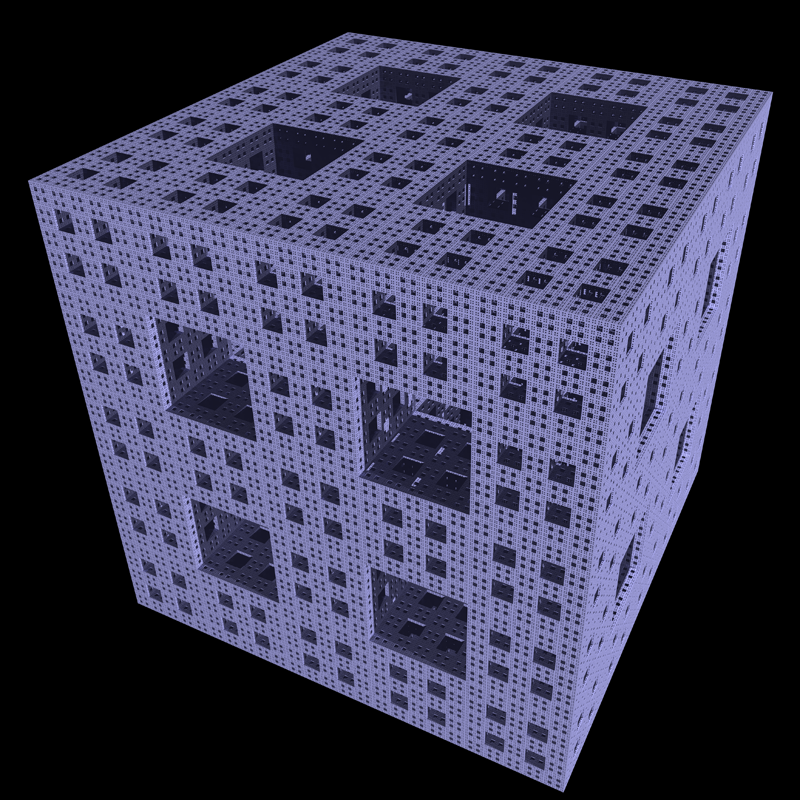The fractal dimension of the mrlysponge5 is log(81)/log(5) = 2.730424777943941.

For those who may want to play along at home, the Povray code that generates iteration 1 from iteration 0 (a cube) is given here. Simply repeat this for subsequent iterations.

mrlysponge7

The first 3 iterations of the mrlysponge7 are as follows.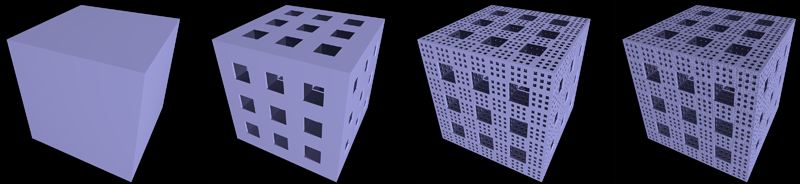A higher resolution version of the 3rd iteration is shown below. At each iteration there are 135 empty spaces and 208 filled spaces. This 3rd iteration then has 2083 ~ 9 million cubes. The next iteration would have about 1.9 billion cubes and is a step to far for the rendering technique used here.
Movie showing generation of the mrlysponge7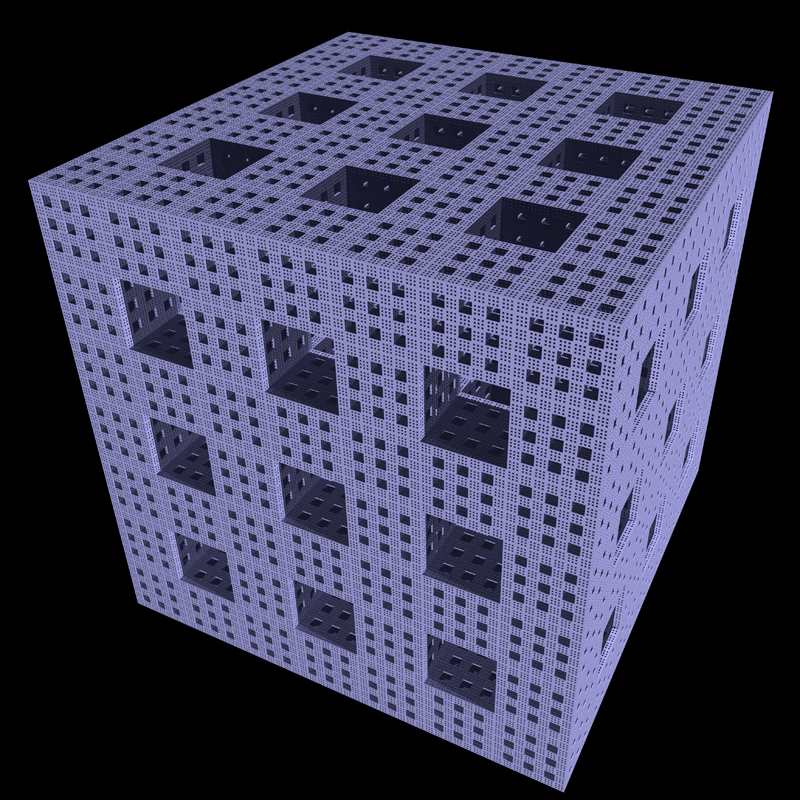The fractal dimension of the mrlysponge7 is log(208)/log(7) = 2.74295197149393.

The Povray code that generates iteration 1 from iteration 0 (a cube) is given here. Simply repeat this for subsequent iterations.

Slices

The internal structure can be revealed by taking various slices through the solid. Some example are given below for mrlysponge5 using a vertical cutting plane, a angled plane and a plane along the diagonal of the cube. This is also a way of creating tiling patterns based upon the mrly sponges.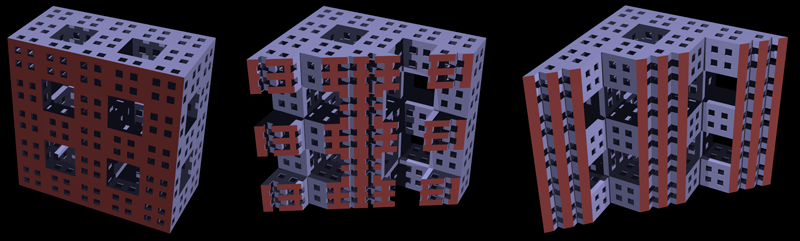Movie showing slicesMovie showing slices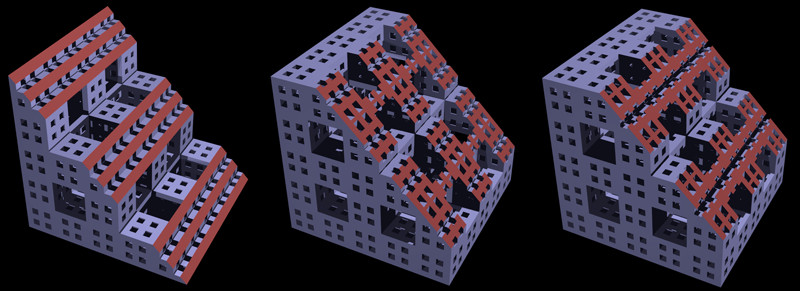Movie showing slices